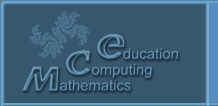Русский
 ! Вопросы и ответы

## Presentations

### Categorical models for neural networks and biomolecules

Tolokonnikov G.K.

FNAT VIM, 109428, RF, Moscow, 1st Institutskiy pr., building 1, office 304

The work belongs to the direction of convolutional polycategories developed by the author , generalizing the polycategories introduced by Szabo in 1975 (see Garner RHG Adv.Math, 2008, 218, 781-827), and the categorical theory of systems built on their basis, generalizing traditional mathematical approaches (M. Mesarovich, V.N. Matrosov, S.N. Vasiliev and others), formalizing the theory of functional systems of P.K. Anokhin. Arrow compositions correspond to convolution in polycategories. For convolutional polycategories, a first-order axiomatics is constructed, similar to that proposed for category theory by Hetcher , substantiating two types of duality, corresponding to an analogue of ordinary duality in category theory, and a new kind of duality, corresponding to permutation of objects and names of polyarrows. Both duality are used in the categorical model of artificial neural networks  to substantiate the well-known intuitive formulas of Osovsky  of the error backpropagation method . The justification is based on the following

Theorem. An artificial neural network is an associative compositional convolutional polycategory with convolutions of the "crown" type.

Convolutional polycategories are built from categorical splices. The Gelman-Feynman theorem makes it possible to construct a categorical model for molecules (convolution corresponds to the joining of atoms into molecules, which is consistent with Bader's approach), to simulate structural chemical formulas, and various types of bonds (hydrogen, aromatic, covalent) corresponding to known approximations of molecular orbitals. Rigorous categorical models for biomolecules, in particular, DNA and RNA, provide for algebraic biology , in addition to the available methods of matrix genetics, a rich categorical algebraic arsenal of research tools, for strictly mathematical prediction of the properties of organisms based on genome analysis.

Literature

1. Tolokonnikov G.K. Informal category theory of systems // Biomashsystems, vol. 2, no. 4, pp. 41-134.

2. Hatcher W.S. The logical foundations of mathematics, Pergamon Press, 1982, 320 p.

3. Osovsky S. Neural networks for information processing, M., 2017, 448s.

4. Tolokonnikov G.K. Convolution Polycategories and Categorical Splices for Modeling Neural Networks. Advances in Intelligent Systems and Computing, Vol. 938, pp. 259-267 (2020).

5. Tolokonnikov G.K., Petoukhov S.V. New mathematical approaches to the problems of algebraic biology, Advances in Intelligent Systems and Computing, Volume 1126, pp. 55-64 (2020).

© 2004 Designed by Lyceum of Informational Technologies №1533